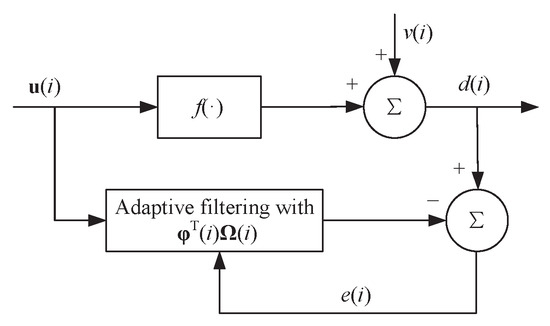Next Article in Journal
Price Movement Prediction of Cryptocurrencies Using Sentiment Analysis and Machine Learning
Next Article in Special Issue
Smooth Function Approximation by Deep Neural Networks with General Activation Functions
Open AccessArticle

Kernel Risk-Sensitive Mean p-Power Error Algorithms for Robust Learning

byTao Zhang 1,2,Shiyuan Wang 1,2,*,Haonan Zhang 1,2,Kui Xiong 1,2 andLin Wang 1,2
1
College of Electronic and Information Engineering, Southwest University, Chongqing 400715, China
2
Chongqing Key Laboratory of Nonlinear Circuits and Intelligent Information Processing, Chongqing 400715, China
*
Author to whom correspondence should be addressed.
Entropy 2019, 21(6), 588; https://doi.org/10.3390/e21060588
Received: 8 May 2019 / Revised: 11 June 2019 / Accepted: 12 June 2019 / Published: 13 June 2019
(This article belongs to the Special Issue Information Theoretic Learning and Kernel Methods)
As a nonlinear similarity measure defined in the reproducing kernel Hilbert space (RKHS), the correntropic loss (C-Loss) has been widely applied in robust learning and signal processing. However, the highly non-convex nature of C-Loss results in performance degradation. To address this issue, a convex kernel risk-sensitive loss (KRL) is proposed to measure the similarity in RKHS, which is the risk-sensitive loss defined as the expectation of an exponential function of the squared estimation error. In this paper, a novel nonlinear similarity measure, namely kernel risk-sensitive mean p-power error (KRP), is proposed by combining the mean p-power error into the KRL, which is a generalization of the KRL measure. The KRP with $p = 2$ reduces to the KRL, and can outperform the KRL when an appropriate p is configured in robust learning. Some properties of KRP are presented for discussion. To improve the robustness of the kernel recursive least squares algorithm (KRLS) and reduce its network size, two robust recursive kernel adaptive filters, namely recursive minimum kernel risk-sensitive mean p-power error algorithm (RMKRP) and its quantized RMKRP (QRMKRP), are proposed in the RKHS under the minimum kernel risk-sensitive mean p-power error (MKRP) criterion, respectively. Monte Carlo simulations are conducted to confirm the superiorities of the proposed RMKRP and its quantized version. View Full-Text
Show FiguresFigure 1

MDPI and ACS Style

Zhang, T.; Wang, S.; Zhang, H.; Xiong, K.; Wang, L. Kernel Risk-Sensitive Mean p-Power Error Algorithms for Robust Learning. Entropy 2019, 21, 588.

Show more citation formats Show less citations formats
Note that from the first issue of 2016, MDPI journals use article numbers instead of page numbers. See further details here.

1